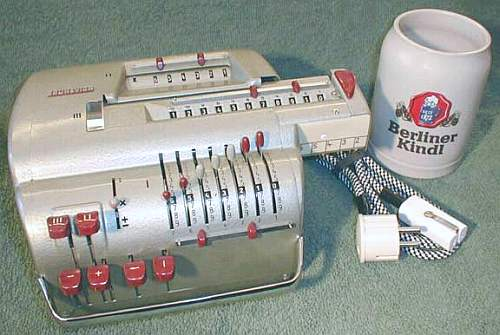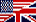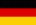previous <<==>> next

BRUNSVIGA-11E----------------------------------------------------------------Original Design - except for the "(self-made) Positioning Scale" on the right of the pulled out Arithmetic-Register (III) Have a look inside ... ========================================================================= TECHNICAL DATA of the Electro-Mechanical Calculator BRUNSVIGA-11E ************************************************************************* Serial-Number: 11-26128 Dimensions: (ca.) Width = 8_1/4" Depth = 9 " Height = 4_1/2" Weight: (ca.) 12 lbs Power Supply: 220 V / 60 W (grounded, AC / DC ) Mechanics: Split-Stepped Drum Functions: Add, Subtract, Multiply, Divide Registers: Input = 7 Decimals Counter = 6 Decimals Arithmetic = 11 Decimals Manufacturer: O L Y M P I A - Werke AG - BRUNSVIGA-Werke - Braunschweig West Germany 1961 BASICS & HOW TO USE the BRUNSVIGA-11E **************************************** KEYS (I), (II), (III) CLEAR the appropriate registers. After clearing the counter (II), a white mark shows its neutral state. It depends of the 1st turn of the Split-Stepped Drum what happens: When (for addition) the 1st turn is clockwise, the counter shows white figures; when (for subtraction) the 1st turn is counter-clockwise, the counter shows red figures. In both cases the counter will work with 10s-carry and allows "Shortened Method of Multiplication". The arithmetic unit (III) can be pulled to the right a maximum of 6 positions. The position of the unit is displayed by a white-red MARK in counter (II). The ARROW-key (<-) moves the arithmetic unit left. The TOGGLE lever accepts 3 positions: +/- = Input register (I) is CLEARED after each ADD/SUB cycle. x = Input register (I) is NOT CLEARED after each ADD/SUB cycle. For MULTIPLICATION, please refer to section MULTIPLY. : = DIVISION (Please refer to section DIVIDE) FUNCTIONS: + = Add Addend + Addend = Sum - = Subtract Minuend - Subtrahend = Difference x = Multiply Multiplicand x Multiplicator = Product : = Divide Dividend : Divisor = Quotient Prior to each task the 3 REGISTERS (I), (II), (III) have to be CLEARED. Register (III) should be at POSITION 1. ADDITION & SUBTRACTION: ----------------------- Example: 123 + 45 - 6 = 162 ADD: Enter the first number (123) in the far right of register (I). Press the PLUS-key (+) to transfer the input (I) into arithmetic unit (III). The counting unit (II) displays the figure 1. Enter the second number (45) into register (I). Press the PLUS key (+) to add the number. The arithmetic unit (III) displays the intermediate sum (168) and the counting unit (II) displays the figure 2. SUBTRACT: Enter the third number (6) into register (I) and press the MINUS-key (-). The arithmetic unit (III) displays the result (162) and the counting unit (II) is decreased by 1. REMARK: NEGATIVE RESULTS are displayed in the arithmetic unit (III) as the COMPLEMENT of the next higher 10, 100, 1000, ... Example: -12 = 99...9988 MULTIPLICATION: --------------- Example: 123 x 45 = 5535 Clear registers (I), (II), (III). Set toggle lever to multiply (x). Enter the multiplicand (123) in the far right of register (I). The multiplicator (45) has two digits, so the arithmetic unit (III) is pulled out to position 2. Repeat pressing the PLUS-key (+), until the first figure of multiplicator (4) will appear in the 2nd position of counter (II). Move the arithmetic unit (III) with the ARROW-key (<-) to position 1. Repeat pressing the PLUS-key (+) until the second figure of the multiplicator (5) appears in the 1st position of counter (II). The multi- plication is done: The multiplicand (123) stays in register (I), the multiplicator (45) is in counter (II) and the result (5535) is in the arithmetic unit (III). DIVISION: --------- Example: 22 : 7 = 3.14285 Remainder 5 Division requires 3 steps: (A) To Set the Dividend into Arithmetic Unit (III): ----------------------------------------------- For the maximum number of decimals, pull out the arithmetic unit (III) to position 6. The toggle lever is either at (+/-) or (x). Enter the dividend (22) in the far right of register (I). Press the PLUS-key (+) to transfer into register (III). (B) To Set the Divisor into Register (I): ------------------------------------- Clear the counter (II) with key (II). Set toggle lever to divide (:). Enter the divisor (7) into register (I) below the dividend (22). (C) To Divide: ---------- Press the MINUS-key (-) and the machine will calculate one decimal and move the arithmetic unit (III) one step to the left. Repeat pressing the MINUS-key (-), until the required number of decimals are calculated... The result (3.14285) can be seen at counter (II) in RED, and the remainder (5) is in the arithmetic unit (III). The divisor (7) is in register (I), therefore an additional decimal can be estimated... ( 7, cause 7 x 7 = 49 ). impressum: ************************************************************************* © C.HAMANN http://public.BHT-Berlin.de/hamann 04/14/07